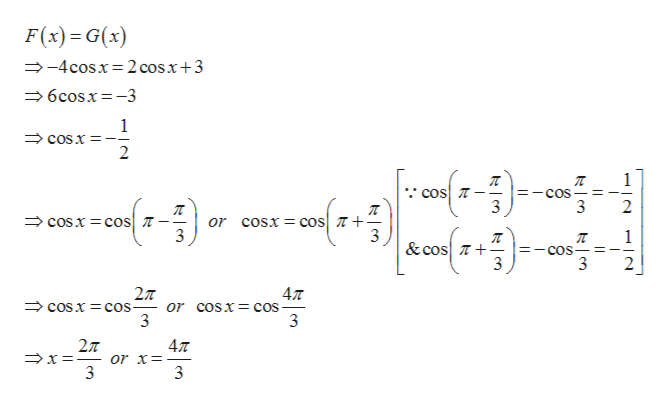# 0<x<2piF(x)=-4cosxG(x)=2cosx+3graph f(x) and g(x) on the same coordinate system for 0<x<2pi and solve f(x)=g(x) on [0,2pi] and label point of intersection on the graph (both x and y values)

Question
1 views

0<x<2pi

F(x)=-4cosx

G(x)=2cosx+3

graph f(x) and g(x) on the same coordinate system for 0<x<2pi and solve f(x)=g(x) on [0,2pi] and label point of intersection on the graph (both x and y values)

check_circle

Step 1

Given,

Step 2

The graph of both the functions is,

Step 3

Now on simplifi...help_outlineImage TranscriptioncloseF(x) G(x) -4cosx 2 cosx+3 6cosx-3 1 cOsx 2 1 cos COS 3 2 cosx cos cosx coS or 3 &cos cos 3 3 2л - or coSX- cos 3 477 COSX cos 3 2T 47T or x=- 3 fullscreen

### Want to see the full answer?

See Solution

#### Want to see this answer and more?

Solutions are written by subject experts who are available 24/7. Questions are typically answered within 1 hour.*

See Solution
*Response times may vary by subject and question.
Tagged in

### Trigonometric Ratios